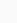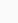# Rbse solutions for Class 8 maths Chapter 1- Rational Numbers

### Class 8 Chapter 1- Rational Numbers Exercise 1.1 Page Number 14

In solutions for Rbse Class 8 maths Chapter 1- Rational Numbers chapter, students will be learning properties related to real numbers, integers, whole numbers, rational numbers and natural numbers – independent, associative and closure. The chapter deals with the role of zero and one, multiplication over addition as well as the representation of Rational Numbers on the number line, along with searching for Rational Numbers between two Rational Numbers. The topics of additive identity (0) and multiplicative identity (1) are also covered in this chapter. There are 2 exercises in this chapter, which contain questions from all the topics present in the chapter.

#### 1. Using appropriate properties find.

(i) -2/3 × 3/5 + 5/2 – 3/5 × 1/6

##### Solution:

-2/3 × 3/5 + 5/2 – 3/5 × 1/6

= -2/3 × 3/5– 3/5 × 1/6+ 5/2 (by commutativity)

= 3/5 (-2/3 – 1/6)+ 5/2

= 3/5 ((- 4 – 1)/6)+ 5/2

= 3/5 ((–5)/6)+ 5/2 (by distributivity)

= – 15 /30 + 5/2

= – 1 /2 + 5/2

= 4/2

= 2

(ii) 2/5 × (- 3/7) – 1/6 × 3/2 + 1/14 × 2/5

Solution:

##### 2/5 × (- 3/7) – 1/6 × 3/2 + 1/14 × 2/5

= 2/5 × (- 3/7) + 1/14 × 2/5 – (1/6 × 3/2) (by commutativity)

= 2/5 × (- 3/7 + 1/14) – 3/12

= 2/5 × ((- 6 + 1)/14) – 3/12

= 2/5 × ((- 5)/14)) – 1/4

= (-10/70) – 1/4

= – 1/7 – 1/4

= (– 4– 7)/28

= – 11/28

2. Write the additive inverse of each of the following

Solution:

(i) 2/8

Additive inverse of 2/8 is – 2/8

(ii) -5/9

Additive inverse of -5/9 is 5/9

(iii) -6/-5 = 6/5

Additive inverse of 6/5 is -6/5

(iv) 2/-9 = -2/9

Additive inverse of -2/9 is 2/9

(v) 19/-16 = -19/16

Additive inverse of -19/16 is 19/16

3. Verify that: -(-x) = x for.

(i) x = 11/15

(ii) x = -13/17

Solution:

(i) x = 11/15

We have, x = 11/15

The additive inverse of x is – x (as x + (-x) = 0)

Then, the additive inverse of 11/15 is – 11/15 (as 11/15 + (-11/15) = 0)

The same equality 11/15 + (-11/15) = 0, shows that the additive inverse of -11/15 is 11/15.

Or, – (-11/15) = 11/15

i.e., -(-x) = x

(ii) -13/17

We have, x = -13/17

The additive inverse of x is – x (as x + (-x) = 0)

Then, the additive inverse of -13/17 is 13/17 (as 11/15 + (-11/15) = 0)

The same equality (-13/17 + 13/17) = 0, shows that the additive inverse of 13/17 is -13/17.

Or, – (13/17) = -13/17,

i.e., -(-x) = x

4. Find the multiplicative inverse of the

(i) -13 (ii) -13/19 (iii) 1/5 (iv) -5/8 × (-3/7) (v) -1 × (-2/5) (vi) -1

Solution:

(i) -13

Multiplicative inverse of -13 is -1/13

(ii) -13/19

Multiplicative inverse of -13/19 is -19/13

(iii) 1/5

Multiplicative inverse of 1/5 is 5

(iv) -5/8 × (-3/7) = 15/56

Multiplicative inverse of 15/56 is 56/15

(v) -1 × (-2/5) = 2/5

Multiplicative inverse of 2/5 is 5/2

(vi) -1

Multiplicative inverse of -1 is -1

5. Name the property under multiplication used in each of the following.

(i) -4/5 × 1 = 1 × (-4/5) = -4/5

(ii) -13/17 × (-2/7) = -2/7 × (-13/17)

(iii) -19/29 × 29/-19 = 1

Solution:

(i) -4/5 × 1 = 1 × (-4/5) = -4/5

Here 1 is the multiplicative identity.

(ii) -13/17 × (-2/7) = -2/7 × (-13/17)

The property of commutativity is used in the equation

(iii) -19/29 × 29/-19 = 1

Multiplicative inverse is the property used in this equation.

6. Multiply 6/13 by the reciprocal of -7/16

Solution:

Reciprocal of -7/16 = 16/-7 = -16/7

According to the question,

6/13 × (Reciprocal of -7/16)

6/13 × (-16/7) = -96/91

7. Tell what property allows you to compute 1/3 × (6 × 4/3) as (1/3 × 6) × 4/3

Solution:

1/3 × (6 × 4/3) = (1/3 × 6) × 4/3

Here, the way in which factors are grouped in a multiplication problem, supposedly, does not change the product. Hence, the Associativity Property is used here.

8. Is 8/9 the multiplication inverse of? Why or why not?

Solution:= -7/8[Multiplicative inverse ⟹ product should be 1]

According to the question,

8/9 × (-7/8) = -7/9 ≠ 1

Therefore, 8/9 is not the multiplicative inverse of.

9. If 0.3 the multiplicative inverse of? Why or why not?

Solution:= 10/3

0.3 = 3/10[Multiplicative inverse ⟹ product should be 1]

According to the question,

3/10 × 10/3 = 1

Therefore, 0.3 is the multiplicative inverse of.

10. Write

(i) The rational number that does not have a reciprocal.

(ii) The rational numbers that are equal to their reciprocals.

(iii) The rational number that is equal to its negative.

Solution:

(i)The rational number that does not have a reciprocal is 0. Reason:

0 = 0/1

Reciprocal of 0 = 1/0, which is not defined.

(ii) The rational numbers that are equal to their reciprocals are 1 and -1.

Reason:

1 = 1/1

Reciprocal of 1 = 1/1 = 1 Similarly, Reciprocal of -1 = – 1

(iii) The rational number that is equal to its negative is 0.

Reason:

Negative of 0=-0=0

11. Fill in the blanks.

(i) Zero has reciprocal.

(ii) The numbers and are their own reciprocals

(iii) The reciprocal of – 5 is .

(iv) Reciprocal of 1/x, where x ≠ 0 is .

(v) The product of two rational numbers is always a .

(vi) The reciprocal of a positive rational number is .

Solution:

(i) Zero has no reciprocal.

(ii) The numbers -1 and 1 are their own reciprocals

(iii) The reciprocal of – 5 is -1/5.

(iv) Reciprocal of 1/x, where x ≠ 0 is x.

(v) The product of two rational numbers is always a rational number.

(vi) The reciprocal of a positive rational number is positive.

### class 8 Chapter 1- Rational Numbers Exercise 1.2 Page Number 20

1. Represent these numbers on the number line.

(i) 7/4

(ii) -5/6

Solution:

(i) 7/4

Divide the line between the whole numbers into 4 parts. i.e., divide the line between 0 and 1 to 4 parts, 1 and 2 to 4 parts and so on.

Thus, the rational number 7/4 lies at a distance of 7 points away from 0 towards positive number line.

(ii) -5/6

Divide the line between the integers into 4 parts. i.e., divide the line between 0 and -1 to 6 parts, -1 and -2 to 6 parts and so on. Here since the numerator is less than denominator, dividing 0 to – 1 into 6 part is sufficient.

Thus, the rational number -5/6 lies at a distance of 5 points, away from 0, towards negative number line

2. Represent -2/11, -5/11, -9/11 on a number line.

Solution:

Divide the line between the integers into 11 parts.

Thus, the rational numbers -2/11, -5/11, -9/11 lies at a distance of 2, 5, 9 points away from 0, towards negative number line respectively.

3. Write five rational numbers which are smaller than 2.

Solution:

The number 2 can be written as 20/10

Hence, we can say that, the five rational numbers which are smaller than 2 are:

2/10, 5/10, 10/10, 15/10, 19/10

4. Find the rational numbers between -2/5 and ½.

Solution:

Let us make the denominators same, say 50.

-2/5 = (-2 × 10)/(5 × 10) = -20/50

½ = (1 × 25)/(2 × 25) = 25/50

Ten rational numbers between -2/5 and ½ = ten rational numbers between -20/50 and 25/50

Therefore, ten rational numbers between -20/50 and 25/50 = -18/50, -15/50, -5/50, -2/50, 4/50, 5/50, 8/50, 12/50, 15/50, 20/50

5. Find five rational numbers between.

(i) 2/3 and 4/5

(ii) -3/2 and 5/3

(iii) ¼ and ½

Solution:

(i) 2/3 and 4/5

Let us make the denominators same, say 60

i.e., 2/3 and 4/5 can be written as:

2/3 = (2 × 20)/(3 × 20) = 40/60

4/5 = (4 × 12)/(5 × 12) = 48/60

Five rational numbers between 2/3 and 4/5 = five rational numbers between 40/60 and 48/60

Therefore, Five rational numbers between 40/60 and 48/60 = 41/60, 42/60, 43/60, 44/60, 45/60

(ii) -3/2 and 5/3

Let us make the denominators same, say 6

i.e., -3/2 and 5/3 can be written as:

-3/2 = (-3 × 3)/(2× 3) = -9/6

5/3 = (5 × 2)/(3 × 2) = 10/6

Five rational numbers between -3/2 and 5/3 = five rational numbers between -9/6 and 10/6

Therefore, Five rational numbers between -9/6 and 10/6 = -1/6, 2/6, 3/6, 4/6, 5/6

(iii) ¼ and ½

Let us make the denominators same, say 24.

i.e., ¼ and ½ can be written as:

¼ = (1 × 6)/(4 × 6) = 6/24

½ = (1 × 12)/(2 × 12) = 12/24

Five rational numbers between ¼ and ½ = five rational numbers between 6/24 and 12/24

Therefore, Five rational numbers between 6/24 and 12/24 = 7/24, 8/24, 9/24, 10/24, 11/24

6. Write five rational numbers greater than -2.

Solution:

-2 can be written as – 20/10

Hence, we can say that, the five rational numbers greater than -2 are

-10/10, -5/10, -1/10, 5/10, 7/10

7. Find ten rational numbers between 3/5 and ¾,

Solution:

Let us make the denominators same, say 80.

3/5 = (3 × 16)/(5× 16) = 48/80

3/4 = (3 × 20)/(4 × 20) = 60/80

Ten rational numbers between 3/5 and ¾ = ten rational numbers between 48/80 and 60/80

Therefore, ten rational numbers between 48/80 and 60/80 = 49/80, 50/80, 51/80, 52/80, 54/80, 55/80, 56/80, 57/80, 58/80, 59/80

Patio umbrellas sold at Costco recalled after reports of fires गर्मी में लू लगने से बचाव करेंगे यह खाद्य पदार्थ, आज ही खाना करें शुरू ट्रेन के बीच में ही AC कोच क्यों लगाए जाते हैं? Rbse books for class 1 to 12 hindi medium 2021-22